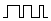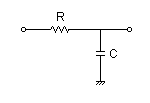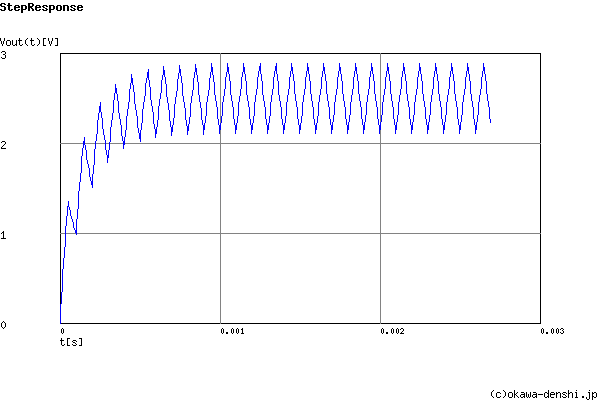# (Sample)RC Low-pass Filter Design for PWM - Result -

Calculated peak-to-peak ripple voltage and settling time at a given PWM frequency and cut-off frequency or values of R and C.

## CR FilterPWM signal →→Vout(s)
(Sample)Transfer Function:
 G(s)= 6283.18530718 s+6283.18530718

fc = 1000[Hz]

g(∞) = 2.5[V]

#### Peak-to-peak ripple voltage

ΔVpk-pk = 0.779001646108[V](Duty=50%)

#### Settling time 0%→90% (0V→2.25V) (without a ripple)

tr = 0.00036646779944[sec]
fPWM=Hz
Duty Step   0%→[%]
PWM signal voltage:
VL = [V]  VH = [V]

### R and C values of filter | Cut-off frequency

Cut-off frequency fc = [Hz]
R and C values
 R=Ω C=F
p:pico, n:nano, u:micro, k:kilo, M:mega

# Transient analysis